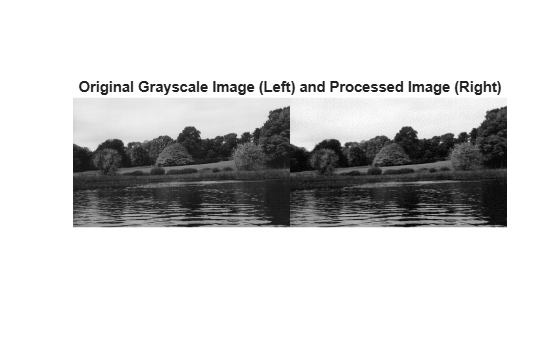dct2

2-D discrete cosine transform

Description

example

B = dct2(A) returns the two-dimensional discrete cosine transform of A. The matrix B contains the discrete cosine transform coefficients B(k1,k2).

B = dct2(A,m,n) and

B = dct2(A,[m n]) pad the matrix A with 0s to size m-by-n before applying the transformation. If m or n is smaller than the corresponding dimension of A, then dct2 crops A before the transformation.

Examples

collapse all

Read an image into the workspace, then convert the image to grayscale.

I = im2gray(RGB);

Perform a 2-D DCT of the grayscale image using the dct2 function.

J = dct2(I);

Display the transformed image using a logarithmic scale. Notice that most of the energy is in the upper left corner.

imshow(log(abs(J)),[])
colormap parula
colorbarSet values less than magnitude 10 in the DCT matrix to zero.

J(abs(J) < 10) = 0;

Reconstruct the image using the inverse DCT function idct2. Rescale the values to the range [0, 1] expected of images of data type double.

K = idct2(J);
K = rescale(K);

Display the original grayscale image alongside the processed image. The processed image has fewer high frequency details, such as in the texture of the trees.

montage({I,K})
title('Original Grayscale Image (Left) and Processed Image (Right)');Input Arguments

collapse all

Input matrix, specified as a 2-D numeric matrix.

Number of image rows, specified as a positive integer. dct2 pads image A with 0s or truncates image A so that it has m rows. By default, m is equal to size(A,1).

Number of image columns, specified as a positive integer. dct2 pads image A with 0s or truncates image A so that it has n columns. By default, n is equal to size(A,2)

Output Arguments

collapse all

Transformed matrix using a two-dimensional discrete cosine transform, returned as an m-by-n numeric matrix.

Data Types: double

collapse all

Discrete Cosine Transform

The discrete cosine transform (DCT) is closely related to the discrete Fourier transform. It is a separable linear transformation; that is, the two-dimensional transform is equivalent to a one-dimensional DCT performed along a single dimension followed by a one-dimensional DCT in the other dimension. The definition of the two-dimensional DCT for an input image A and output image B is

where

and

M and N are the row and column size of A, respectively.

Tips

• If you apply the DCT to real data, the result is also real. The DCT tends to concentrate information, making it useful for image compression applications.

• To invert the DCT transformation, use idct2.

 Jain, Anil K., Fundamentals of Digital Image Processing, Englewood Cliffs, NJ, Prentice Hall, 1989, pp. 150–153.

 Pennebaker, William B., and Joan L. Mitchell, JPEG: Still Image Data Compression Standard, Van Nostrand Reinhold, 1993.